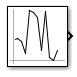# Random Number

Generate normally distributed random numbers

• Library:## Description

The Random Number block generates normally distributed random numbers. To generate uniformly distributed random numbers, use the Uniform Random Number block.

You can generate a repeatable sequence using any Random Number block with the same nonnegative seed and parameters. The seed resets to the specified value each time a simulation starts. By default, the block produces a sequence that has a mean of 0 and a variance of 1. To generate a vector of random numbers with the same mean and variance, specify the Seed parameter as a vector.

Avoid integrating a random signal, because solvers must integrate relatively smooth signals. Instead, use the Band-Limited White Noise block.

The numeric parameters of this block must have the same dimensions after scalar expansion. If you select the Interpret vector parameters as 1-D check box, and the numeric parameters are row or column vectors after scalar expansion, the block outputs a 1-D signal. If you clear the Interpret vector parameters as 1-D check box, the block outputs a signal of the same dimensionality as the parameters.

## Ports

### Output

expand all

Output signal that is the generated random numbers falling within a normal Gaussian distribution. The output is repeatable for a given seed.

Data Types: `double`

## Parameters

expand all

Specify the mean of the random numbers generated.

#### Programmatic Use

 Block Parameter: `Mean` Type: character vector Values: scalar Default: `'0'`

Specify the variance of the random numbers.

#### Programmatic Use

 Block Parameter: `Variance` Type: character vector Values: scalar Default: `'1'`

Specify the starting seed for the random number generator.

The output of number generated is repeatable for a given seed.

#### Programmatic Use

 Block Parameter: `Seed` Type: character vector Values: scalar Default: `'0'`

Specify the time interval between samples. The default is `0.1`, which matches the default sample time of the Band-Limited White Noise block. See Specify Sample Time in the Simulink® documentation for more information.

#### Programmatic Use

 Block Parameter: `SampleTime` Type: character vector Values: scalar Default: `'0.1'`

Select this check box to output a vector of length `N` if the Constant value parameter evaluates to an `N`-element row or column vector.

• When you select this check box, the block outputs a vector of length `N` if the Constant value parameter evaluates to an `N`-element row or column vector. For example, the block outputs a matrix of dimension `1-by-N` or `N-by-1`.

• When you clear this check box, the block does not output a vector of length `N` if the Constant value parameter evaluates to an `N`-element row or column vector.

#### Programmatic Use

 Block Parameter: `VectorParams1D` Type: character vector Values: `'on' | 'off'` Default: `'on'`

## Block Characteristics

 Data Types `double` Direct Feedthrough `no` Multidimensional Signals `yes` Variable-Size Signals `no` Zero-Crossing Detection `no`